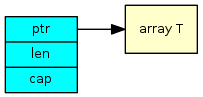# Go basics - slices

## Definition

A slice is a structure holding a pointer to an array of elements of a certain type as well as a capacity and a length that respectively represent the total number of elements that the array can contain and its present usage.## Lifecycle

A slice is initialized by calling `make()`. This invocation takes either two or three arguments depending on the equality between the slice length and capacity. The first argument indicates the type of the underlying array. The second argument represent the slice desired length and the last argument its capacity, but is optional if the same as the length.

Depending on the machine architecture the maximum slice capacity may vary. The Go runtime will check for the requested size when calling `make()` and panic if it exceeds the max allocatable size for the current architecture.

Architecture  Maximum slice capacity
MIPS 2.00 GiB
32-bit 3.99 GiB
64-bit 256 TiB

The `len()` function returns the slice length and `cap()` returns the slice capacity.

### Declaration

``````var slice []int
``````
###### Pitfalls
• An unitialized slice is equivalent to a zero capacity slice. Indexing such a slice will trigger a panic.

### Initialization

``````// Slice of int with a length of 2 elements and a capacity of 10 elements.
slice = make([]int, 2, 10)

// l equals 2
l := len(slice)

// c equals 10
c := cap(slice)

// Slice of int with a length and a capacity of 5 elements.
slice = make([]int, 5)
``````

## Manipulation

#### Select

Selecting a subset of a slice is done by using the notation `[i:j]`. If `i` is omitted, it is implicitely set to the index of the first element of the slice. Similarly, if `j` is omitted, it is implicitely set to the index of the last element of the slice plus one.

The range is inclusive on the left index and exclusive on the right index.

``````slice = make([]int, 10)

// elements at indexes 2 to 9
subslice = slice[2:]

// elements at indexes 0 to 6
subslice = slice[:7]

// elements at indexes 2 to 6
subslice = slice[2:7]
``````

#### Append

All append operations are handled by calling `append()`.

``````// newSlice holds all elements of slice and "1", "2" at the end
newSlice = append(slice, 1, 2)

// Append elements of otherSlice to slice
slice = append(slice, otherSlice...)

// Delete element at index 3
slice = append(slice[:3], slice[4:]...)
``````
###### Pitfalls
• When `cap(slice)-len(slice)` is smaller than the set of elements to append, a new slice is created with at least the capacity for all elements to fit in. The new slice capacity depends on the old slice characteristics. Three situations can arise:
• The resulting size exceeds the double of the old slice capacity: it is allocated just the necessary capacity for all elements to fit in.
• Else, if the length of the old slice is not greater than 1023: it is allocated a capacity corresponding to twice the capacity of the old slice.
• Eventually, if the length of the old slice is greater than 1023: the new capacity will be computed by iteratively increasing the capacity of the old slice by 25% until it exceeds the exact size required for all elements to fit in. This last value becomes the capacity of the new slice.

#### Copy

``````itemCount := copy(destination, source)
``````
###### Pitfalls
• The amount of elements copied from the source slice to the destination slice is equal to the lowest length of the two.

That’s it for Go slices. I hope it was informative!